# NOT Gate: What is it? (Working Principle & Circuit Diagram)

Contents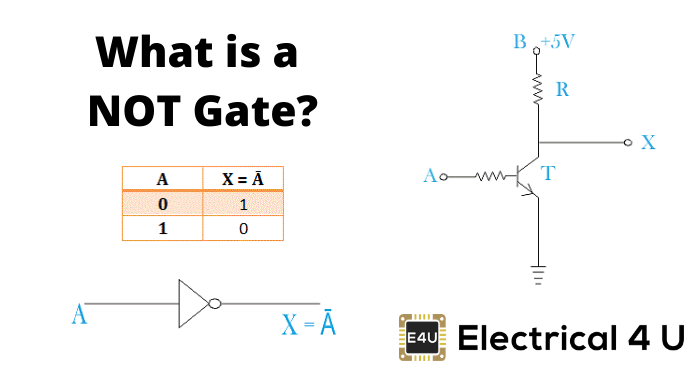## What is a NOT Gate?

NOT gate is a logic gate that inverts the digital input signal. For this reason, a NOT gate is sometimes is referred to as an inverter (not to be confused with a power inverter). A NOT gate always has high (logical 1) output when its input is low (logical 0). Conversely, a logical NOT gate always has low (logical 0) output when the input is high (logical 1). The logical symbol for a NOT gate is shown below: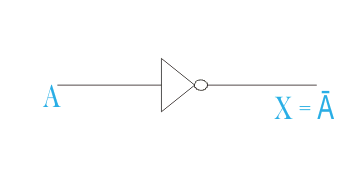If the input binary variable of a NOT gate is considered as A, then the output binary variable of the gate will be Ā. As the symbol of not operation is ( – ) bar. If the value of A is 1.
then Ā = 0 and in the opposite if the value of A is then Ā = 1.

Truth tables list the output of a particular digital logic circuit for all the possible combinations of its inputs. The truth table of a NOT gate can be represented as: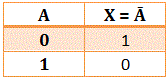Other logic gates include AND gates, OR gates, NAND gates, NOR gates, XOR gates, XNOR gates.

## NOT Gate Transistor Circuit Diagram

A NOT gate can easily be realized by using a simple bipolar transistor. The transistor circuit diagram of a NOT gate (also known as a transistor inverter) is shown below: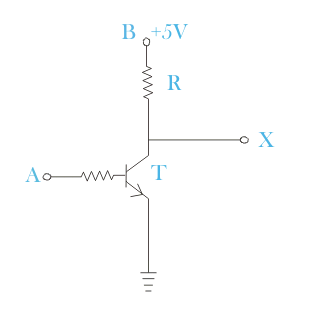The transistor diagram above will be used to demonstrate how a NOTE gate works.

## How a NOT Gate Works

Let us examine the above transistor circuit when a high input voltage is applied, i.e. +5V.In this condition, the transistor T gets enough base potential to make it ‘ON’.

As soon as the transistor becomes ON, the supply voltage (+5V) at B will get a path to the earth through the resistor R. At ON condition the transistor will behave short-circuited ideally, hence entire supply voltage will drop across resistor R and no voltage will appear at X and hence the output of the inverter or NOT gate will be zero. In actual fact, there will be some voltage drop across the collector and emitter even at ON condition, of the transistor.

This collector-emitter voltage is about 0.6V. So, at the above-said input condition, the entire supply voltage +5V will not drop across the resistor instead it will be 5 – 0.6 = 4.4V. So, 0.6V is practically considered as logical zero or low.

Now let us examine the condition, where input A = 0V i.e. base terminal of the transistor is given with 0V or grounded.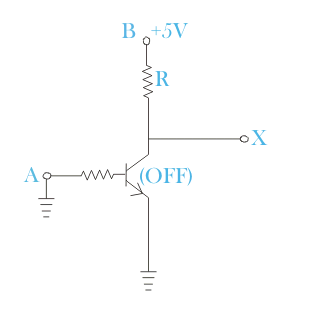At that condition, as the base of the transistor is at 0 potential, the transistor T will be in OFF condition and hence, the supply voltage will not get any path to the earth and the entire supply voltage will appear at the output terminal of the NOT gate high or logical 1, when input terminal A is low or logical zero.

## NOT Gate IC

The IC available at the market for NOT gate is IC 7404. One 7404 IC contains a total of six transistors inverter or simply six NOT gate.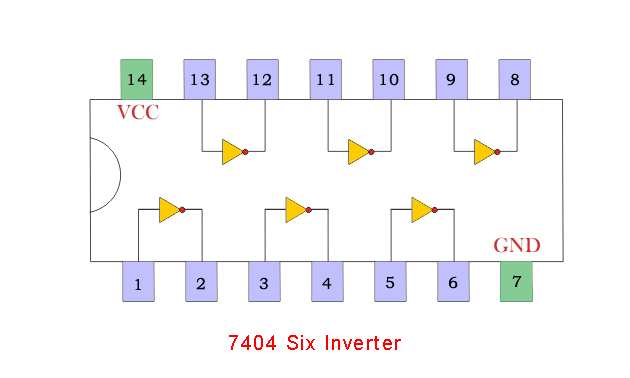Want To Learn Faster? 🎓
Get electrical articles delivered to your inbox every week.
No credit card required—it’s 100% free.